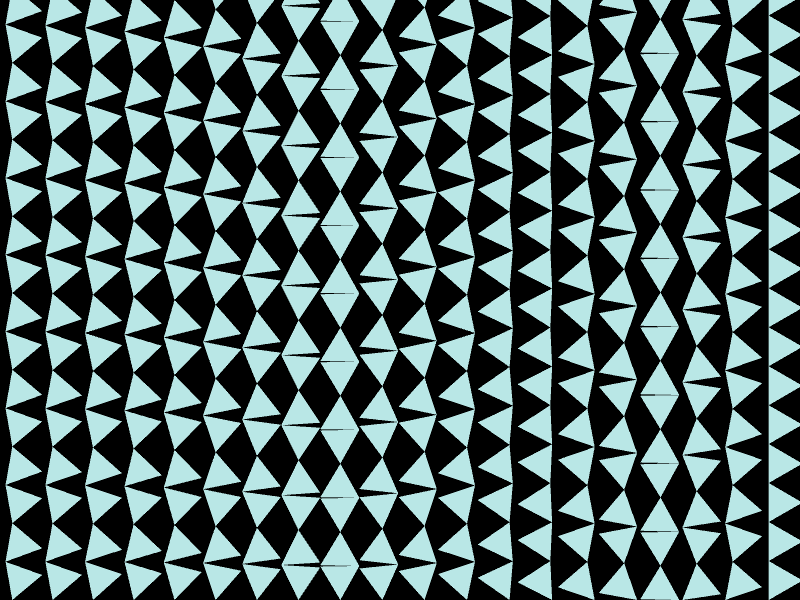• Savehttps://www.dwitter.net/d/15095

Javascript...

H=c.height|=0;p=2*Math.PI/3;for(i=23;Y=H,i--;x.fill(),t+=i/599)for(x.beginPath(k=16);k--;t=-t)for(o=48*C((m=((t+p/4)%p+p)%p)>p/2?p-m:m),Y-=(Y==H?1:2)*o,j=7;j--;x.arc(i*83+48,Y,48,q=t+j*p,q));

384 views

keyboard shortcuts: L or F like